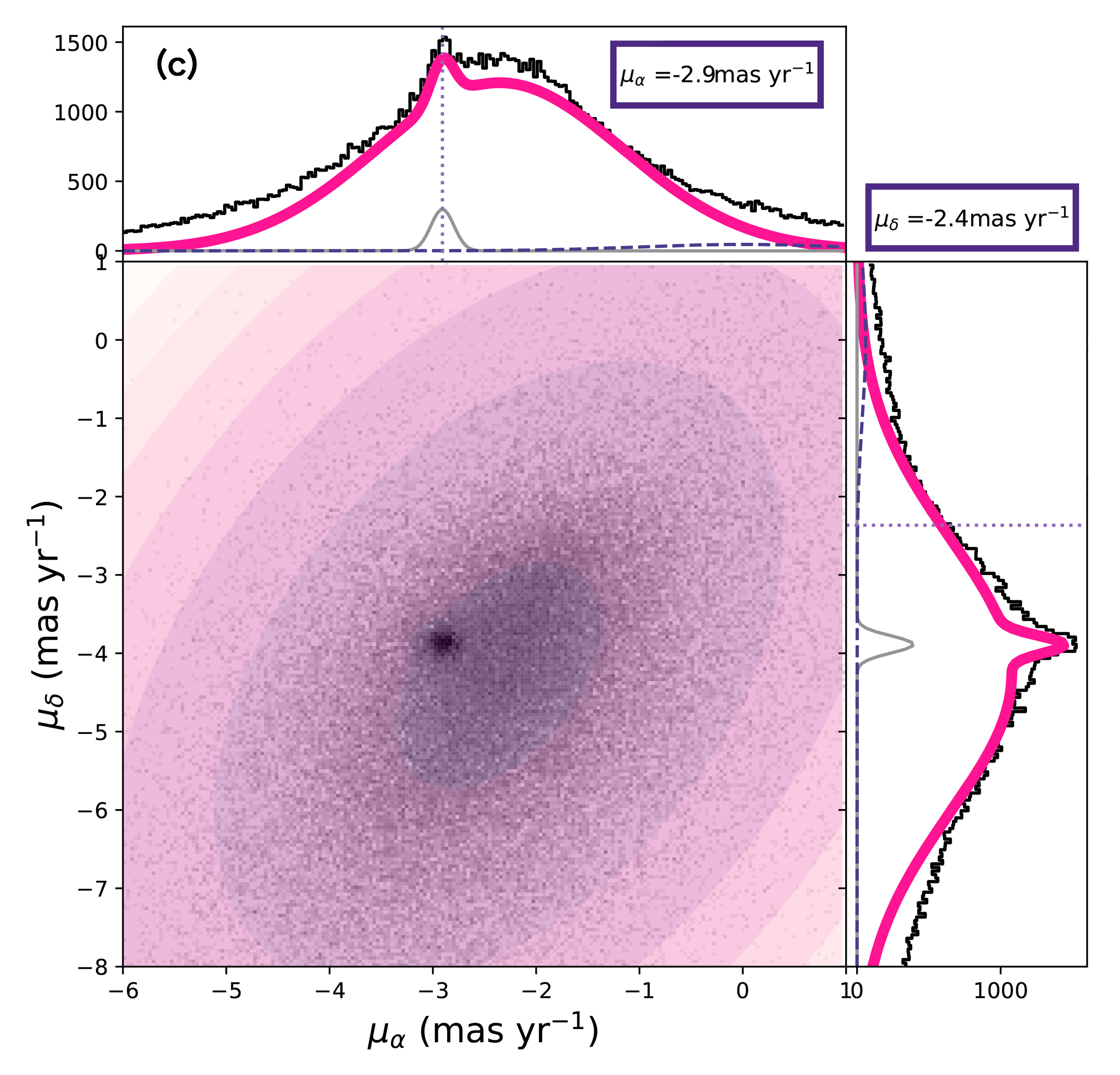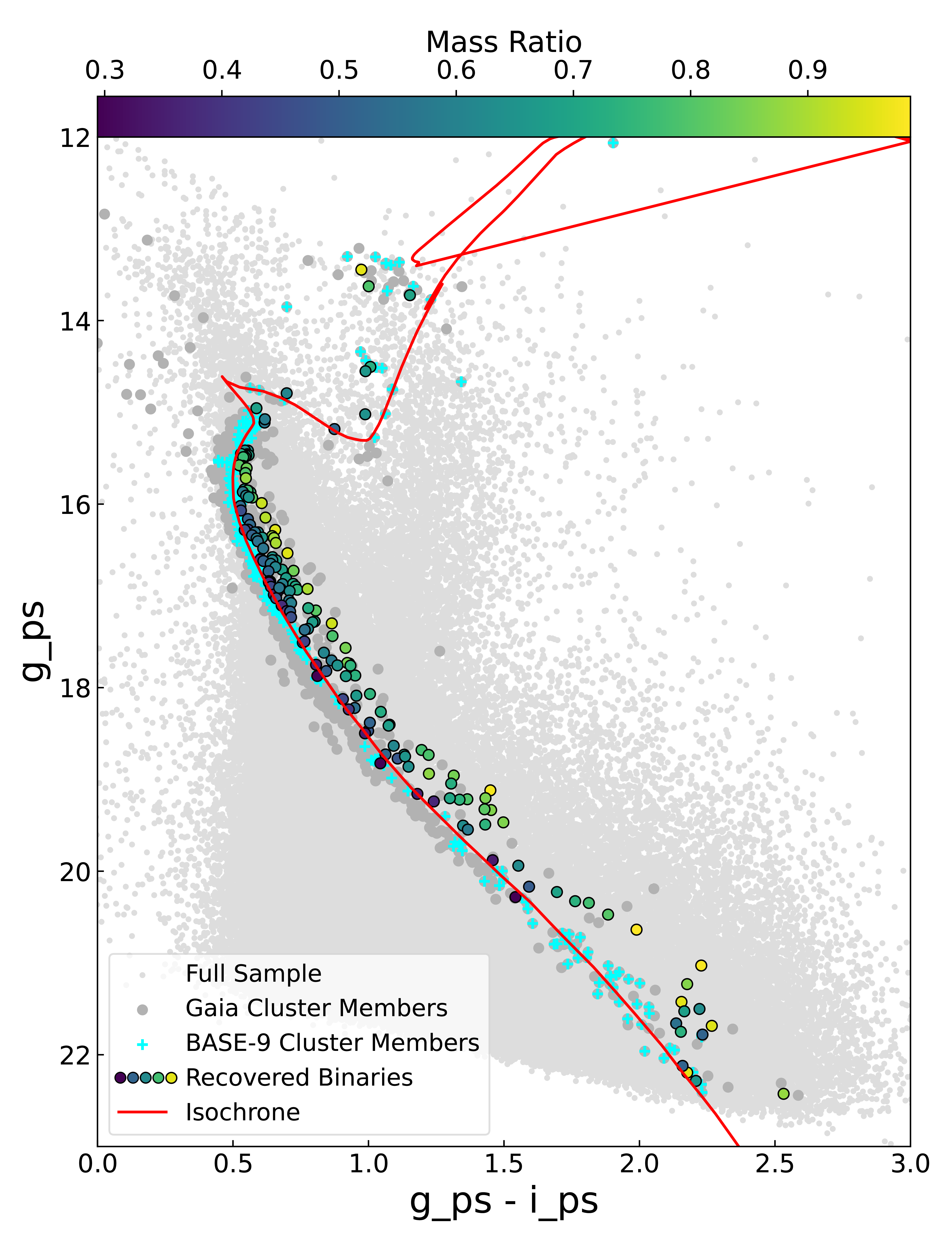Applying Bayesian Statistics to
Identify Binaries in NGC 6819

Claire Zwicker, Illinois Institute of Technology
CIERA REU at Northwestern University

# BACKGROUND

NGC 6819 is a loose region of stars that are gravitationally bound to each other. Within NGC 6819 are several hundred cluster members, which all have similar age, distance, metallicity, and reddening. Many binaries are thought to form during star and consequently cluster formation. In star clusters, close gravitational encounters can also form new binaries, destroy binaries and exchange stars in/out of binaries. Binaries help us understand star formation, stellar evolution pathways, and how a cluster will evolve dynamically over time.

We can apply a tool called Bayesian Analysis for Stellar Evolution with Nine Parameters (BASE-9) to inspect the evolution of clusters and gain insight into the qualities of it such as age, distance modulus, metallicity, and reddening.

NGC 6819 is reported to have an age of 2.4 $$\pm$$ .02 Gyr, a distance modulus of 12.22 $$\pm$$ .1 mag, a metallicity of .051 $$\pm$$ .02 dex, and a reddening of E(B-V) = 0.130 $$\pm$$ 0.035 mag (Ak et al. 2016); (Milliman et al. 2014). NGC 6819 has been previously reported to have a parallax distance around 2309 $$\pm$$ 106 pc (Ak et al. 2016) and 2.82 kpc (Yang et al. 2022), a radial velocity around 2.34 $$\pm$$ 0.05 km/s (Hole et al. 2009) and 2.4 km/s (Milliman et al. 2014), and proper motions of <$$\mu_{\alpha}$$> = -2.916 mas/yr and <$$\mu_{\delta}$$> = -3.866 mas/yr (Yang et al. 2022).

# METHODOLOGY

## How We Filtered Out Nonmembers Before Using BASE-9

We first identified probable cluster members using astrometric data available in the Gaia, 2MASS, and Pan-STARRS photometric surveys. One of the challenges in doing so is that field stars were present between our line of sight to the cluster, as well as behind the cluster. We recovered members using the cluster's radial velocity, parallax, and proper motion, since those parameters are distinct from surrounding field stars. Gaia supplies this data, and after plotting histograms and fitting the distributions, the member probabilities can then be calculated with the equation below.Figure 1.Membership probability calculation from Geller et al.Figure 2. The Gaussian fits and corresponding cluster values for (a) radial velocity in km/s (RV), (b) distance in pc, and (c) proper motions in mas/yr.

Multiplying those three probabilities together provides an overall probability, and we chose a desired minimum for membership.

# RESULTSFigure 3. A color magnitude diagram of NGC 6819, depicting the binaries that were recovered from our cluster sample using Gaia photometry. An isochrone from BASE-9 is plotted, along with a colorbar showing the mass ratios for each binary. Figure 4 (above). A histogram of NGC 6819's mass ratios found using BASE-9. Binaries that lie closer to the isochrone have lower mass ratios, making it difficult to assess whether a binary has been detected. As a result, binary stars with a mass ratio of q < 0.5 are left incomplete.

## BASE-9 Identified 169 Binaries in NGC 6819

We selected the photometry of these cluster members and used BASE-9's singlePopMcmc feature. This sampled each of the cluster's parameters (age, distance modulus, metallicity, and reddening) with a Markov chain Monte Carlo algorithm. We input values for these parameters that we believed were accurate, known as the priors; these priors informed the algorithm where to sample. They were fed into singlePopMcmc alongside the photometry file, which then ran thousands of iterations to sample each parameter space. This required many computing hours to complete, which was leveraged with Northwestern's Quest High Performance Computing Cluster.

Once the script finished running, we were left with thousands of data points, from which we constructed a histogram and fit it with a Gaussian for the most probable value. As a result, the cluster's age, distance modulus, metallicity, and reddening was found. When the cluster's age was determined, we used another feature of BASE-9 called makeCMD in order to generate a mean fit isochrone. These techniques have been applied before with NGC 188 described by Cohen et al. (2020).

The derived cluster parameters were used along with the photometry in BASE-9's sampleMass feature to sample potential binaries. This feature outputs two columns for each cluster member; a potential primary star mass and its ratio between the secondary. It does so for hundreds of iterations, sampling potential combinations for each star. Once the script finished, the primary masses and mass ratios are averaged for each object. If an object's secondary mass was greater than $$3\sigma$$ from the mean, we considered it to be a binary. Since computing time on Quest was limited to 1 week (168 hours total), we ran sampleMass in parallel to reduce overall processing time.Figure 5. Sampling plot and histograms of the posterior distributions for the cluster parameters derived by BASE-9 for NGC 6819. The mean and standard deviation is also shown on the right for each distribution, for reference.

We found that singlePopMcmc had poor sampling when faint stars were included in the run. To remedy this we removed stars fainter than 17 mag from the photometry file prior to running. We also found that including more than one survey's filters would similarly sample poorly. Pan-STARRS filters were chosen when deriving the cluster members; Gaia and 2MASS weren't included. The cluster parameters found from this trimmed photometry file were then used with the original photometry file within sampleMass.

## Conclusion and Future Improvements

We used BASE-9 and identified 169 binaries in the open cluster NGC 6819. This translates to a binary fraction of approximately 34.9$$\%$$ $$\pm$$ 2.69$$\%$$. A previous study reported "a incompleteness-corrected binary fraction for all MS binaries with periods less than 104 days to be 22$$\%$$ $$\pm$$ 3$$\%$$" (Milliman et al. 2014). We also derived the following cluster parameters: a log(age) of 9.462 $$\pm$$ .0012, a metallicity of -.109 $$\pm$$ .0043 dex, a distance modulus of 12.231 $$\pm$$ .0057, and a reddening of 505.84 $$\pm$$ 2.78 mmag.

These values have corresponding errors that are precise, which demonstrates the power and utility of a Bayesian statistics approach. Yet, these results rely on the accuracy of the models which BASE-9 utilizes, which means these error constraints may not be nearly as precise as we may think.

NGC 6819's isochrone did not fit the main sequence as anticipated; it lies slightly to the right for some of the Gaia Cluster Members in Figure 3. Ideally, it would encompass those cluster members more closely. Future work and improvements with sampling NGC 6819 in singlePopMcmc is needed. This involves including more filters than just Pan-STARRS for singlePopMcmc. Furthermore, mass ratios of q < 0.5 are incomplete, and will need to be corrected.

BASE-9 is capable of predicting several parameters of a cluster. We hope to be able to apply the techniques outlined to many clusters. Automation of these operations will enable us to examine trends across many clusters, which will prove useful for future stellar astrophysics work. Future work is necessary to explore the accuracy of the models BASE-9 uses and the extent of its features as well.

## References

Ak, T., Bostanci, Z. F., Yontan, T., et al. 2016, Ap&SS, 361, 126
Cohen, R. E., Geller, A. M., & von Hippel, T. 2020, AJ, 159, 11
Geller, A. M., Mathieu, R. D., Harris, H. C., & McClure, R. D. 2008, AJ, 135, 2264
Hole, K. T., Geller, A. M., Mathieu, R. D., et al. 2009, The Astronomical Journal, 138, 159.
Milliman, K. E., Mathieu, R. D., Geller, A. M., et al. 2014, AJ, 148, 38
von Hippel, T., Robinson, E., Jeffery, E., et al. 2014, arXiv e-prints, arXiv:1411.3786
Yang, Y., Li, C., Huang, Y., & Liu, X. 2022, The Astrophysical Journal, 925, 159.